# geometryFromEdges

Create 2-D geometry from decomposed geometry matrix

## Syntax

``geometryFromEdges(model,g)``
``pg = geometryFromEdges(model,g)``

## Description

example

````geometryFromEdges(model,g)` adds the 2-D geometry described in `g` to the `model` container.```
````pg = geometryFromEdges(model,g)` additionally returns the geometry to the Workspace.```

## Examples

collapse all

Create a decomposed solid geometry model representing a rectangle and include it in a PDE model.

Create a default scalar PDE model.

`model = createpde;`

Define a geometry representing a rectangle.

`R1 = [3,4,-1,1,1,-1,0.5,0.5,-0.75,-0.75]';`

Decompose the geometry into minimal regions.

`g = decsg(R1);`

Create the geometry from the decomposed geometry matrix.

`geometryFromEdges(model,g);`

Plot the geometry.

```pdegplot(model,EdgeLabels="on") axis equal xlim([-1.1,1.1]) ylim([-0.9,0.6])```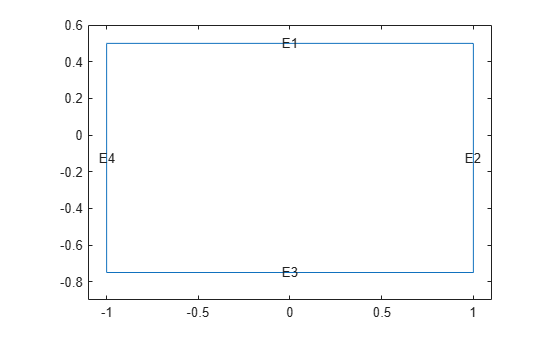Create a decomposed solid geometry model and include it in a PDE model.

Create a default scalar PDE model.

`model = createpde;`

Define a circle in a rectangle, place these in one matrix, and create a set formula that subtracts the circle from the rectangle.

```R1 = [3,4,-1,1,1,-1,0.5,0.5,-0.75,-0.75]'; C1 = [1,0.5,-0.25,0.25]'; C1 = [C1;zeros(length(R1) - length(C1),1)]; gm = [R1,C1]; sf = 'R1-C1';```

Create the geometry.

```ns = char('R1','C1'); ns = ns'; g = decsg(gm,sf,ns);```

Include the geometry in the model and plot it.

```geometryFromEdges(model,g); pdegplot(model,EdgeLabels="on") axis equal xlim([-1.1,1.1])```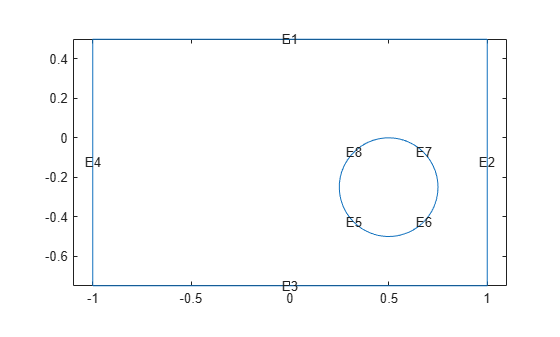The toolbox provides the several geometry functions. Specify them by using the following function handles.

```model = createpde; g = geometryFromEdges(model,@cardg); pdegplot(model)```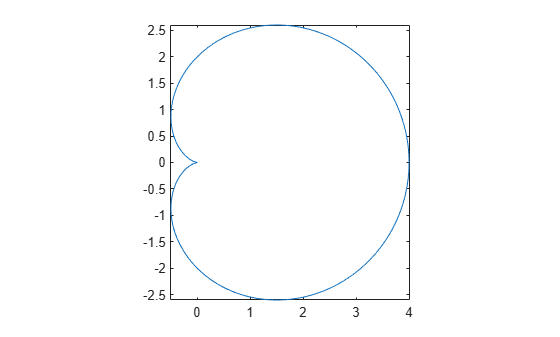```clear model model = createpde; g = geometryFromEdges(model,@circleg); pdegplot(model)```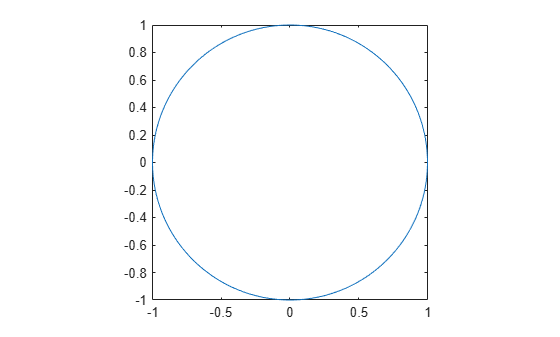```clear model model = createpde; g = geometryFromEdges(model,@cirsg); pdegplot(model)```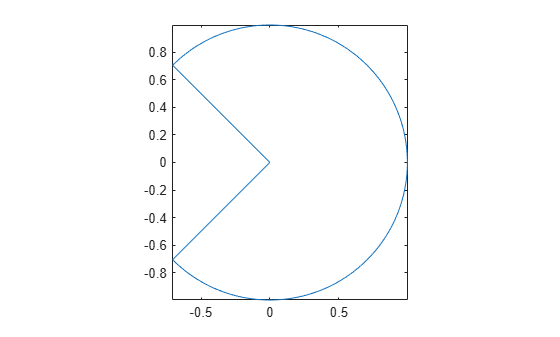```clear model model = createpde; g = geometryFromEdges(model,@crackg); pdegplot(model)```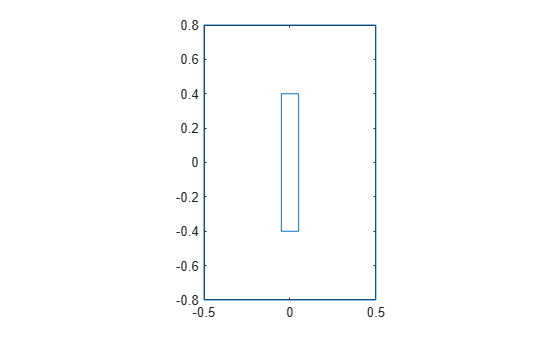```clear model model = createpde; g = geometryFromEdges(model,@lshapeg); pdegplot(model)```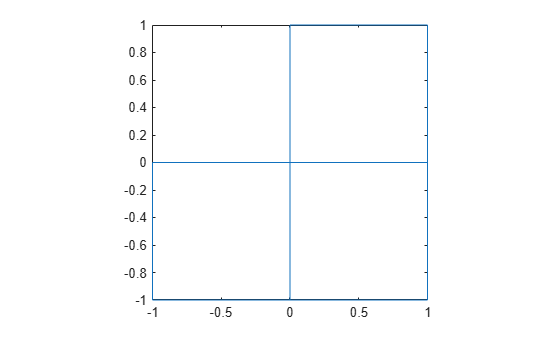```clear model model = createpde; g = geometryFromEdges(model,@scatterg); pdegplot(model)```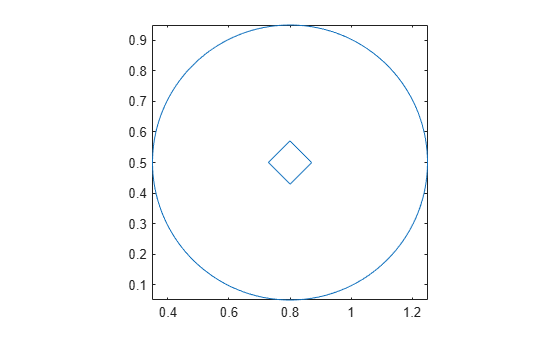```clear model model = createpde; g = geometryFromEdges(model,@squareg); pdegplot(model)```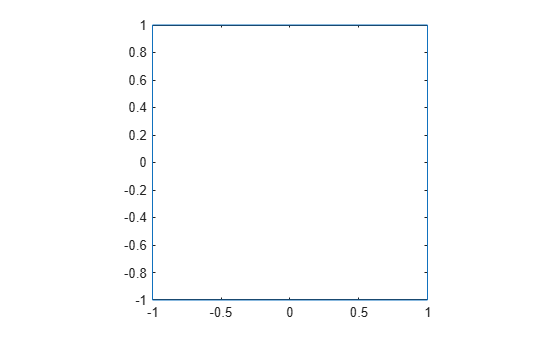## Input Arguments

collapse all

Model container, specified as a `PDEModel` object, `ThermalModel` object, `StructuralModel` object, or `ElectromagneticModel` object.

Example: `model = createpde(3)`

Example: ```thermalmodel = createpde(thermal="steadystate")```

Example: ```structuralmodel = createpde(structural="static-solid")```

Example: ```emagmodel = createpde(electromagnetic="electrostatic")```

Geometry description, specified as a decomposed geometry matrix, as the name of a geometry function, or as a handle to a geometry function. For details about a decomposed geometry matrix, see `decsg`.

A geometry function must return the same result for the same input arguments in every function call. Thus, it must not contain functions and expressions designed to return a variety of results, such as random number generators.

Example: `geometryFromEdges(model,@circleg)`

Data Types: `double` | `char` | `function_handle`

## Output Arguments

collapse all

Geometry object, returned as an AnalyticGeometry Properties object. This object is stored in `model.Geometry`.

## Version History

Introduced in R2015a